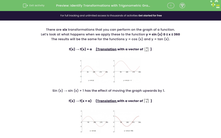# Identify Transformations with Trigonometric Graphs

In this worksheet, students will practise applying the six function transformations to trigonometric graphs.Key stage:  KS 4

Year:  GCSE

GCSE Subjects:   Maths

GCSE Boards:   AQA, Eduqas, OCR, Pearson Edexcel,

Curriculum topic:   Algebra, Graphs of Equations and Functions

Curriculum subtopic:   Graphs Transformation of Curves and Their Equations

Difficulty level:#### Worksheet Overview

There are six transformations that you can perform on the graph of a function.

Let’s look at what happens when we apply these to the function y = sin (x) 0 ≤ x ≤ 360

The results will be the same for the functions y = cos (x) and y = tan (x).

f(x) → f(x) + a     {Translation with a vector of}Sin (x) → sin (x) + 1 has the effect of moving the graph upwards by 1.

f(x) → f(x + a)     {Translation with a vector of}Sin (x) → sin (x + 90) has the effect of moving the graph left by 90°.

This transforms y = sin(x) into y = cos(x).

f(x) → af(x)         {Stretch in the y-axis, scale factor a}Sin (x) → 2sin (x) has the effect of increasing every value of y by 2. It stretches the graph vertically.

f(x) → f(ax)         {Stretch in the x-axis, scale factor 1/a}Sin (x) → sin (2x) has the effect of multiplying every value of x by 1/2. It compresses the graph horizontally.

f(x) → -f(x)          {Reflection in the x-axis }Sin (x) → -sin (x) has the effect of reflecting the entire graph in the x-axis.

f(x) → f(-x)          {Reflection in the y-axis}Sin (x) → sin (-x) has the effect of reflecting the entire graph in the y-axis.

In the case of transforming sin (x), you have probably noticed that sin (-x) = - sin(x).

Now it's time for some questions!

### What is EdPlace?

We're your National Curriculum aligned online education content provider helping each child succeed in English, maths and science from year 1 to GCSE. With an EdPlace account you’ll be able to track and measure progress, helping each child achieve their best. We build confidence and attainment by personalising each child’s learning at a level that suits them.

Get started